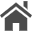Home  Updated: November 15, 2020  [日本語 / English]

# MQL4 Script to Save MT4's Order History to CSV file

## Introduction

I created a MQL4 script that saves MT4's order history to a CSV file that can be easily handled by a programming language such as Python or a spreadsheet software such as Excel.

The output text file is in the following format.The first line is a header (comment), and the order history follows after the second line.

Each line is separated by commas and includes the open time, close time, number of lots, profit and loss, symbol name, and order type.

``````OpenTime,CloseTime,Lots,Profit,Symbol,Buy/Sell
2020.10.26 03:54:05,2020.10.26 04:10:03,1.41,-4371,USDJPY-cd,Sell
2020.10.26 10:36:15,2020.10.26 10:56:00,1.4,3360,USDJPY-cd,Sell
``````

## Program

The program I wrote in MQL4 is as follows. When you execute this as a script on MT4, a text file (CSV format) with a file name of OrderHistory.csv will be output.

The file is saved in the MQL4\files folder in the MT4's "Data Folder". You can open the "Data Folder" by clicking "File" -> "Open Data Folder" on the menu of MT4.

``````#property strict

// Order struct
struct ORDER
{
ORDER():openTime(0.0), closeTime(0.0), lots(0.0), profit(0.0) // Initialize member variables
{
}
datetime openTime;   // Open Time
datetime closeTime;  // Close Time
double lots;         // Number of Lots
double profit;       // Profit and Loss
string symbol;       // Symbol (Currency Pair) Name
};

// Swap ORDER struct
void Swap(ORDER &a, ORDER &b)
{
ORDER c = a;
a = b;
b = c;
}

// Sort the array of ORDER struct in ascending order of Open Time
// Bubble sort!
void Sort(ORDER &a[])
{
for(int i=0 ; i < ArraySize(a) - 1 ; i++)
{
for(int j = ArraySize(a) - 1 ; j > i ; j--)
{
if(a[j].openTime < a[j - 1].openTime) // If you want to change the sorting conditions, change here
{
Swap(a[j], a[j - 1]);
}
}
}
}

// Entry point for MT4 script
void OnStart()
{
// Only output order history from this date and time
const MqlDateTime dt = { 2020, 1, 1, 0, 0, 0 };
const datetime StartTime = StructToTime(dt);

// Total number of orders
const int n = OrdersHistoryTotal();

// Declare an array of OREDE struct and allocate the elements
ORDER orders[];
ArrayResize(orders, n);

// Loop the number of orders
for (int i = 0 ; i < n ; i++)
{
// Select the i-th order in the order history
OrderSelect(i, SELECT_BY_POS, MODE_HISTORY);

// Retrieve data for executed orders only
if (OrderType() == OP_BUY || OrderType() == OP_SELL)
{
orders[i].openTime = OrderOpenTime();
orders[i].closeTime = OrderCloseTime();
orders[i].lots = OrderLots();
orders[i].profit = OrderProfit();
orders[i].symbol = OrderSymbol();
}
}

// Sorting
Sort(orders);

// Open the file
int file = FileOpen("OrderHistory.csv", FILE_CSV | FILE_ANSI | FILE_WRITE, ',');

FileWrite(file, "OpenTime", "CloseTime", "Lots", "Profit", "Symbol", "Buy/Sell");

// Loop the number of orders
for (int i  = 0 ; i < n ; i++)
{
// Only orders opened after the specified date and time are output to the file
if (orders[i].openTime >= StartTime)
{
FileWrite(
file, orders[i].openTime, orders[i].closeTime,
orders[i].lots,orders[i].profit,